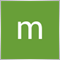# why doesnt this simple loop work?240

``` for( int n=0; n<total; n++ ){
if(OrderMagicNumber()!=333 && number_of_buys == 7) OrderSend(Symbol(),OP_SELL,50,Bid,3,0,0,"hedged",333,0,clrRed);

}```

What should be happening is that the loop scans for trades by magic number and if there are no current trades with the magic number 333 and there are 7 buys, then it places one 50 lot sell trade. The vice versa is true for sell.

However what does happen is that it places infinite 50 lot trades until it crashes. It should only place a 50 lot trade if the conditions are true.

Any idea guys?Moderator
23449

Your loop doesn't check that there are no current trades with the magic number, it checks if the selected order does not have the magic number.

Where are the values for number_of_buys and  number_of_sells calculated?1191

marth tanaka:

...
Any idea guys?

My personal impression is that this loop is - most of the time it is called - about to head into something very, very evil...240

Keith Watford:

Your loop doesn't check that there are no current trades with the magic number, it checks if the selected order does not have the magic number.

Where are the values for number_of_buys and  number_of_sells calculated?

Ohhhhhhhhh I see. So my loop only selects ONE order and sees if it has no magic number = to the one I want and thats why it places so many trades.

Well the fix then would be to make it go through all open orders, make sure THEY dont have the magic number 333 for example and then place a trade. How would I do this? I dont know how to write a loop that would make sure there isnt a magic number in all the open trades before performing an action.

```int _get_number_of_trades(int ttype){ //function that returns number of currently opene trades on current pair
int tCount = 0;

for(int cnt=0;cnt<OrdersTotal();cnt++){

if(OrderSymbol() == Symbol() && OrderType() == ttype) tCount++;
}

return(tCount);
}

```Moderator
23449

```
if(OrderMagicNumber == MagicNumber && OrderSymbol() == Symbol() && OrderType() == ttype)
tCount++;```240

Keith Watford:
This is what I have now (Still doesnt work maybe you can see why)

```int _get_number_of_trades(int ttype){ //function that returns number of currently opene trades on current pair
int tCount = 0;

for(int cnt=0;cnt<OrdersTotal();cnt++){

if(OrderSymbol() == Symbol() && OrderType() == ttype && OrderMagicNumber()!=333||334) tCount++;
}

return(tCount);
}

int tCount = 0;

for(int cnt=0;cnt<OrdersTotal();cnt++){

if(OrderMagicNumber() == 333 && OrderSymbol() == Symbol() && OrderType() == ttype)
tCount++;
}

return(tCount);
}

int _get_number_of_trades_hedge_sell(int ttype){ //function that returns number of currently opene trades on current pair
int tCount = 0;

for(int cnt=0;cnt<OrdersTotal();cnt++){

if(OrderMagicNumber() == 334 && OrderSymbol() == Symbol() && OrderType() == ttype)
tCount++;
}

return(tCount);
}
void hedger()
{

{OrderSend(Symbol(),OP_SELL,50,Bid,3,0,0,"hedged",333,0,clrRed);}
if (sell_trades_number == 7 && numberofhedgedsells == 0)

}```
hedger() runs in my onTick() function. Essentially it checks if there are 7 current buys or sells in and if there are 7 buys in, for example, it should place a 50 lot sell trade. Vice versa.

However it doesnt. It still places multiple and I cant see where Im going wrong.Moderator
23449

`if(OrderSymbol() == Symbol() && OrderType() == ttype && OrderMagicNumber()!=333||334) tCount++;`

Why are you using !=  ??

`if(OrderSymbol() == Symbol() && OrderType() == ttype && (OrderMagicNumber()==333 || OrderMagicNumber()==334)) tCount++;`

Is that what you intended?240

Keith Watford:

Why are you using !=  ??

Is that what you intended?

I am using != in the first function to make sure I dont pick up any trades from prexising hedges before I count all my "normal non 50 lot buy trades".

Basically, if I have 7 other trades (not the 50 lot ones), I want to place a 50 lot trade in the other directionModerator
23449

Then you probably intended

`if(OrderSymbol() == Symbol() && OrderType() == ttype && OrderMagicNumber()!=333 && OrderMagicNumber()!=334) tCount++;`